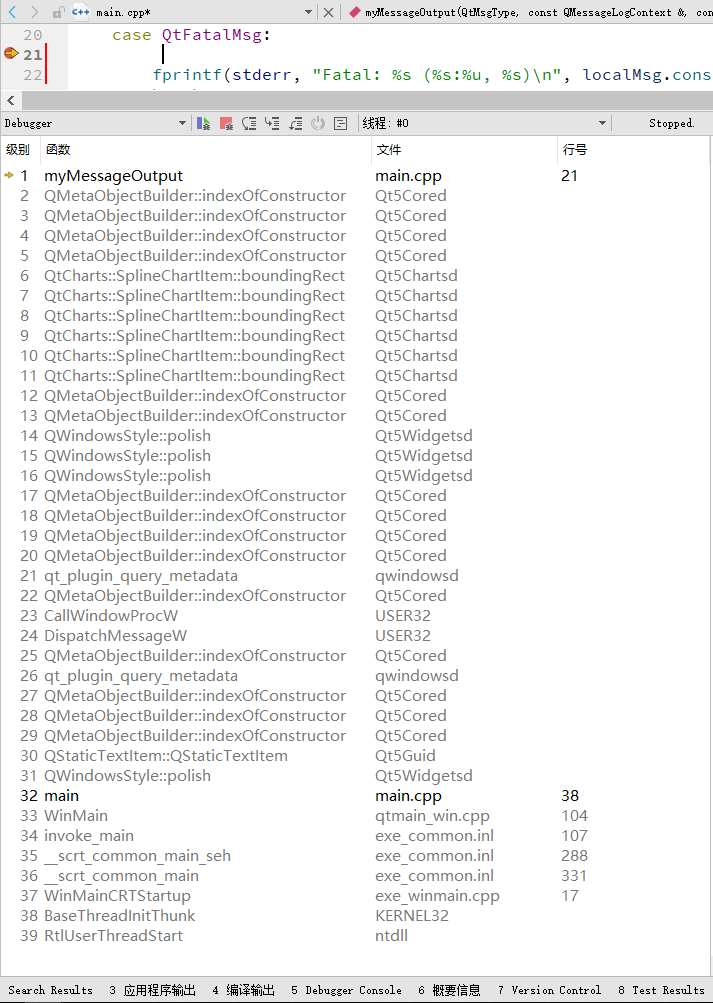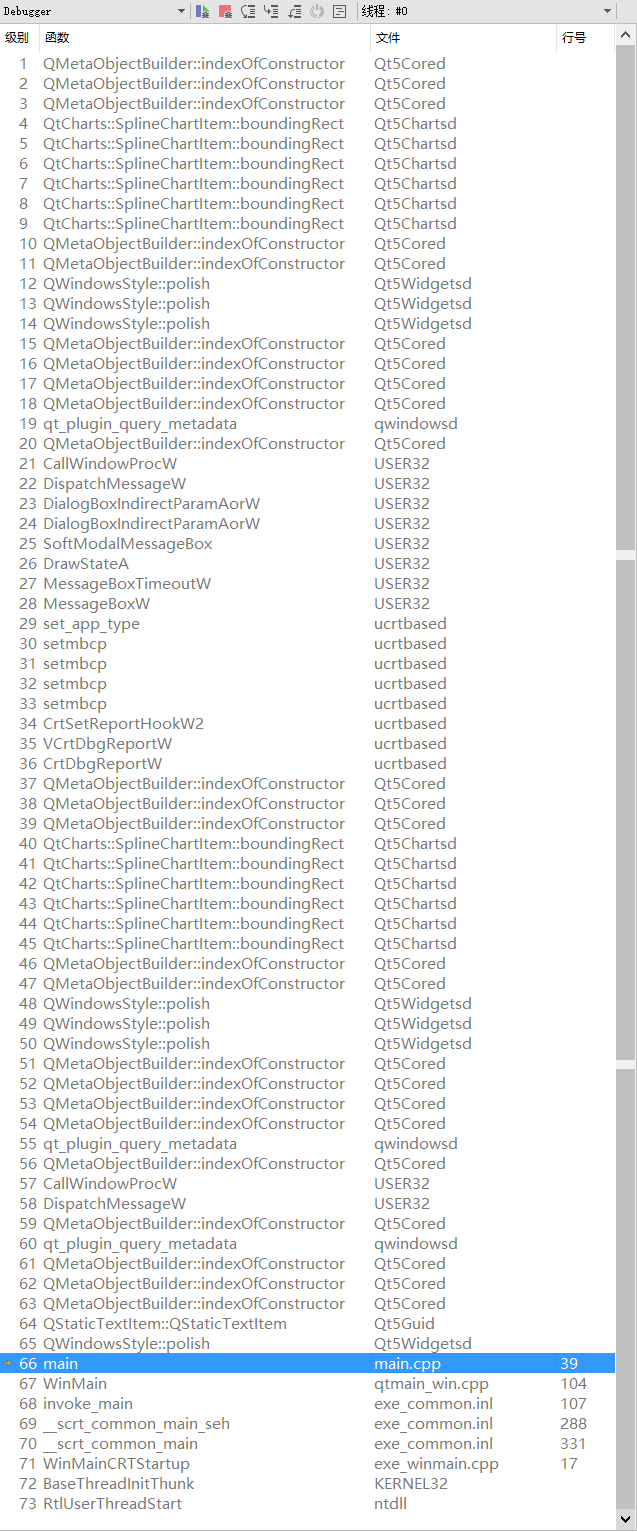# QVector Assert Debug - "asize >= 0 && asize <= aalloc"

• Hello guys, I encounter a problem that is difficult to deal with and need your help. But first of all, let me roughly explain the app I'm working on.

I am working on a little app that reads data from the serial port and then writes them to a local file. At the same time, I need to read the latest certain amount of data from the file (the same local file), and display them on the QChart in the MainWindow.

Assuming the main GUI is executing in Thread 0, the serial part of code is wrapped in a QObject-SerialObj and runs in Thread 1, and the reader part of code is wrapped in a QObject-ReaderObj and runs in Thread 2. Also, there is a ScopeChart object inherited from QChart running in Thread 0 in the MainWindow.

Generally, the approach I'm using now is as following:

• Read data from the serial port and write them to a local file in a fixed time interval controlled by a QTimer in the SerialObj. {Step 1}

• As soon as writing to the local file is finished, SerialObj emits a signal to ReaderObj, which will read a certain amount of the latest data from the file and save them to its member variable-m_wavesData. {Step 2}
(I use a local variable here to read the data and then use "swap" function upon m_wavesData and the local variable. both of them are `QVector<QVector<QPointF>>` ).

• Separately, the ScopeChart will emit &QGraphicsScene::changed signal to a slot in ReaderObj to UPDATE the series in the chart, which will then emit &QGraphicsScene::changed signal again and form a forever loop to update the chart continuously. {Step 3}
(The pointer to the series in the chart is passed to ReaderObj at the beginning. the type of which is `*QVector<QPointF>`. So in the UPDATE, I just assign m_wavesData to the pointer)

However I'm getting this strange error every now and so, saying that:

``````ASSERT: "asize >= 0 && asize <= aalloc" in file c:\Users\qt\work\install\include\QtCore/qvector.h, line 553
``````

I captured this error by using `qInstallMessageHandler`, because I want to debug the Q_ASSERT error. However I don't understand what the debugger stack tells me when I put a breakpoint inside my error handler function. The stack looks like this:Or this:main.cpp line 38 is

``````return app.exec();
``````

So I have no idea where the error occurs. I guess it occurs in {Step 2} while I‘m reading the latest data from the file and updating m_wavesData. But I cannot figure out what's wrong.

I would be very appreciative if anyone have any idea what could cause this and suggestions on my coding or debugging.

PS: My situation is similar to https://forum.qt.io/topic/74846/qvector-size-assert but I found no help from that post.

``````#ifndef READEROBJ_H
#include <QObject>
#include <QPointer>
#include <QPointF>
#include <QVector>
#include <QFile>
#include <QTimer>
#include <QLineSeries>
#include <QMutex>

{
Q_OBJECT
public:
const int &wave_num = 1,
const int &data_length = 100,
const QVector<bool> &wave_table = QVector<bool>(10, true),
QObject *parent = nullptr);
void updateDataSelection(const int &pos, const int &state);

signals:
void info(const QString& body, const QString &head = "INFO");
void warning(const QString& body, const QString &head = "WARNING");
void error(const QString& body, const QString &head = "ERROR");

public slots:
void prepareToUpdate(const QString&);
void updateDataFromFile();
void setDataLength(const int &value){
m_dataLength = value;
}
void updateDataToScope(QtCharts::QLineSeries *);
private:
QPointer<QFile> m_logFile;
int m_waveNum;
int m_dataLength;
QVector<bool> *m_dataSelection = nullptr;
QTimer *m_dataUpdater = nullptr;
QVector<QVector<QPointF>> m_wavesData;
QMutex m_mutex;
};

``````
``````#include "readerobj.h"
#include <QDateTime>
#include <QVector>
#include <QDataStream>
#include <QDebug>
#include <QPoint>

const int &wave_num,
const int &data_length,
const QVector<bool> &wave_table,
QObject *parent) :
QObject(parent),
m_waveNum(wave_num),
m_dataLength(data_length),
m_dataSelection(new QVector<bool>(wave_table)),
m_dataUpdater(new QTimer(this))
{
m_wavesData.reserve(m_waveNum);
for(int i = 0; i < m_waveNum; i++){
QPointF point(20, 20);
QVector<QPointF> v_point(100, point);
//v_point.append(point);
m_wavesData.append(v_point);
}
}
if(!m_logFile.isNull()){
m_logFile->close();
if(!m_logFile->isOpen()){
emit info("Log File Closed Successfully for Reading", "SYSTEM");
}
delete m_logFile;
}
if(m_dataSelection != nullptr){
delete m_dataSelection;
m_dataSelection = nullptr;
}
if(m_dataUpdater != nullptr){
delete m_dataUpdater;
m_dataUpdater = nullptr;
}
.arg(__FUNCTION__)
.arg(__FILE__)
}

m_logFile = new QFile(log_dir);
emit error(QString("Fail to Open Log File to Read. Error Code: %1").arg(m_logFile->error()));
} else{
emit info("Log File Open Successfully for Reading", "SYSTEM");
}
}

qint64 end_index = m_logFile->size();
qint64 total_data = m_waveNum*m_dataLength;
float *f_temp = new float[static_cast<quint32>(total_data)];
if(end_index > 0){
if(end_index >= total_data*4){
m_logFile->seek(end_index - total_data*4);
}
else{
m_logFile->seek(0);
}
QVector<QVector<QPointF>> waves_data;
waves_data.reserve(m_waveNum);
for(int wave_ind = 0; wave_ind < m_waveNum; wave_ind++){
QVector<QPointF> series_data;
for(int data_ind = 0; data_ind < data_read; data_ind++){
series_data.append(QPointF(data_ind, static_cast<double>(*(f_temp + data_ind*m_waveNum + wave_ind))));
}
waves_data.append(series_data);
}
m_mutex.lock();
m_wavesData.swap(waves_data);
m_mutex.unlock();
}
if(f_temp != nullptr){
delete[] f_temp;
f_temp = nullptr;
}
}

for(int i = 0; i < m_waveNum; i++){
if((*m_dataSelection)[i]){
target_series[i].replace(m_wavesData[i]);
}
}
}

void ReaderObj::updateDataSelection(const int &pos, const int &state){
if(state == 2){
(*m_dataSelection)[pos] = true;
} else{
(*m_dataSelection)[pos] = false;
}
}
``````

• It seems that a QMutex locker on the `swap()` function solves this problem.

Sadly, nope. However the error probability is much smaller. I have updated my ReaderObj code above.

• @Secant

Probably the easiest is writing a small wrapper class which inherits from QVector and you overload the access operators in question.
This way you may add some additional output code for display and add also a breakpoint in the debugger.

• @koahnig
Thank you, I will have a try.
I have another small question to ask: What if the QTimer timeout handler slot function consumes too much time longer than the QTimer interval? Will it cause a crash?

• @Secant

• What if the QTimer timeout handler slot function consumes too much time longer than the QTimer interval

That is more likely to just hang the app ( make it slow to respond )
However, it any of the other code access an index that would normally be filled
at that point in time, it could indirectly lead to crash due to altered timing.

• I have another small question to ask: What if the QTimer timeout handler slot function consumes too much time longer than the QTimer interval? Will it cause a crash?

No. You'll get the next timer event at the next possible moment (i.e. when you return control to the event loop). You are also going to get only one timer event.

• Thanks for your explanation. Finally I figured out that the problem lay in the signal `QGraphicsScene::changed` I used. I learned about this signal in an OpenGL example in Qt. By using it I was trying to update my wave soon after its last update, however this signal could also be triggered by changing axis range or window resize, which would probably cause the crash for I didn't consider thread safety and synchronization under this condition.

So I defined a custom signal function and now the problem is solved.